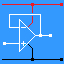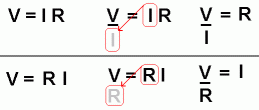RANDOM PAGE

SITE SEARCH

LOG
IN

HELP

# Basic Maths

This is the AQA version closing after June 2019. Visit the the version for Eduqas instead.

a

## Rounding off Numbers

Rounding off to Whole Numbers - Examples

• 2.00001 becomes 2
• 2.4999 becomes 2
• 2.5 becomes 3
• 2.999 becomes 3
b

## BODMAS or BIDMAS

• Brackets, Order, Division, Multiplication, Addition, Subtraction
• Brackets, Index, Division, Multiplication, Addition, Subtraction

This is all about the order in which you do a calculation.

• B        Anything inside brackets is always worked out first.
• O or I        This is for items like Xy or 103 where y and 3 are the order or index. These are worked out after dealing with anything inside brackets.
• D        Division and ...
• M        ... multiplication are done next.
• S        ... and subtraction are done.

For example here are two bits of arithmetic with different meanings ...

3 + 2 x 7        =        17        Do the multiplication first and then add three.

(3 + 2) x 7        =        35        The brackets override the rule above so work out the brackets first and then multiply by seven.

c

## Times Tables

 X 1 2 3 4 5 6 7 8 9 10 11 12 1 1 2 3 4 5 6 7 8 9 10 11 12 2 2 4 6 8 10 12 14 16 18 20 22 24 3 3 6 9 12 15 18 21 24 27 30 33 36 4 4 8 12 16 20 24 28 32 36 40 44 48 5 5 10 15 20 25 30 35 40 45 50 55 60 6 6 12 18 24 30 36 42 48 54 60 66 72 7 7 14 21 28 35 42 49 56 63 70 77 84 8 8 16 24 32 40 48 56 64 72 80 88 96 9 9 18 27 36 45 54 63 72 81 90 99 108 10 10 20 30 40 50 60 70 80 90 100 110 120 11 11 22 33 44 55 66 77 88 99 110 121 132 12 12 24 36 48 60 72 84 98 108 120 132 144
d

## Fractions

Calculate 1/4 + 1/7

Using a calculator with brackets you would enter this ...

1 / 4 + 1 / 7 =

You don't need brackets because the BODMAS rules work without them.

e

## Harder Fractions

Calculate Rt if the formula is this: 1 / Rt = 1 / R1 + 1 / R2

Using a calculator with brackets you would calculate Rt like this ...

1 / ( 1 / R1 + 1 / R2 ) =

f

## Rearranging Formulas

If there are no + or - signs in the formula, re-arranging is easy. Diagonal moves are allowed across the equals sign. This works for most of the formulas for Electronics AS and A2.

Here is an example ...g

## Powers of Ten

Examples - The powers add up.

103 x 104 = 107

10-3 x 104 = 10

100 = 1

10-3 x 10-2 = 10-5

1 / 103 = 10-3

h

## Powers of Ten on a Calculator

There seem to be several methods used by different calculator manufacturers to solve powers of 10. Most students should use a low cost scientific calculator. More advanced models can be too hard to use and sometimes are quite non-standard.

Find out how to enter powers of 10 into your calculator. The most obvious way is nearly always wrong! Look for the Exp button. This is the "Times-ten-to-the" button. Look for the +/- button. This is used to enter things like 10-9. If you use the normal minus button, on many calculators, it won't work. On some calculators the Exp button is labelled x10

i

## Example Calculator Key Presses

If the frequency in Hertz = 1 / (2 π R C), to the nearest Hertz, calculate the frequency if R = 84 kilohms and C = 3 nanofarads.

84 kilohms = 84 x 103 Ohms

Here are the calculator key presses for simple scientific calculators ...

1 / ( 2 x PI x 84 Exp 3 x 3 Exp 9 +/- ) =

Your calculator may be different. Learn how to use it! Get help if you need it!

reviseOmatic V3     Contacts, ©, Cookies, Data Protection and Disclaimers Hosted at linode.com, London